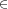## Chapter 42Homework for the fourteenth week

### 42.1 Homework

Notes also available as PDF.

Practice is absolutely critical in this class.

Groups are fine, turn in your own work. Homework is due in or before class on Mondays.

• Exercises for 7.3
• 25, 26, 68, 70
• Exercises for 7.4
• 64, 65, 66 (note: making a profit implies R - C > 0 where R is the revenue and C is the cost)
• Exercises in 7.5
• 60, two different ways. First, substitute points into {x}^{2} + {(x + 30)}^{2} - 15{0}^{2} and plot the line segments. Try x\{80,85,90,95,100\}. In this case, you’ll happen to find the answer. For the other way, use the Pythagorean theorm as in the text.
• Exercises in 8.1
• 56: Use the point formula for a line,  {x - {x}_{0}\over { x}_{1} - {x}_{0}} = {y - {y}_{0}\over { y}_{1} - {y}_{0}},

to determine the equation of the closest points to each requested x. Then substitute the x value in the middle and find the y.

• Exercises in 8.3:
• 70, 72, 74
• Exercises in 8.6:
• 50
• Exercises in 8.7:
• Use either substitution or elimination: 50, 78 (yes, I had to assign a “speed of a train leaving\mathop{\mathop{…}}” problem), 86
• Exercises in 8.8:
• 30, 34

Note that you may email homework. However, I don’t use MicrosoftTM products (e.g. Word), and software packages are notoriously finicky about translating mathematics.

If you’re typing it (which I advise just for practice in whatever tools you use), you likely want to turn in a printout. If you do want to email your submission, please produce a PDF or PostScript document.